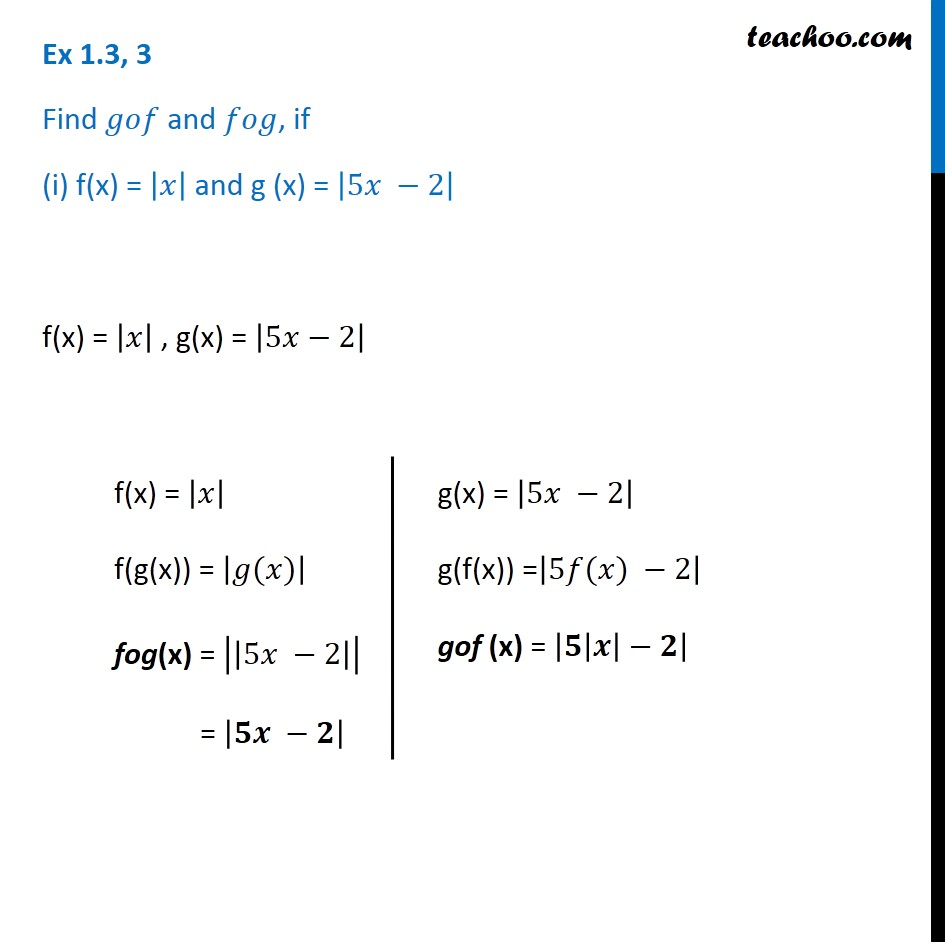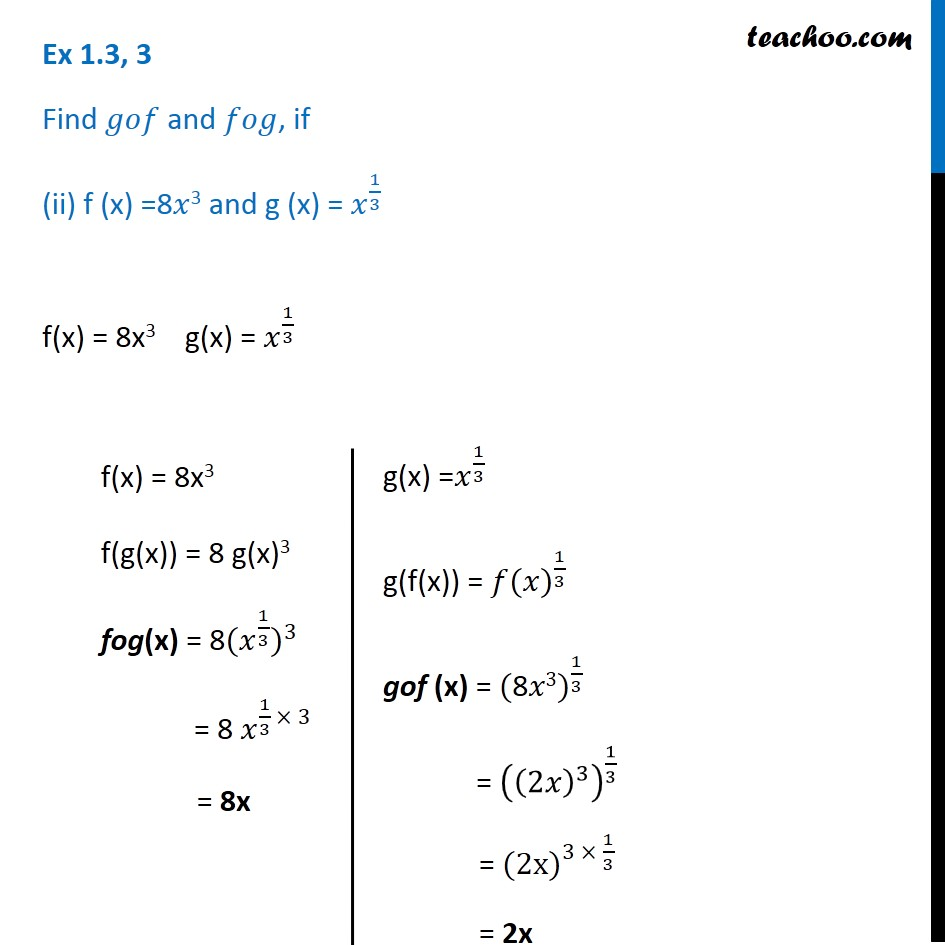Subscribe to our Youtube Channel - https://you.tube/teachoo

1. Chapter 1 Class 12 Relation and Functions
2. Serial order wise
3. Ex 1.3

Transcript

Ex 1.3, 3 Find 𝑔𝑜𝑓 and 𝑓𝑜𝑔, if (i) f(x) = |𝑥| and g (x) = |5𝑥 −2| f(x) = |𝑥| , g(x) = |5𝑥−2| f(x) = |𝑥| f(g(x)) = |𝑔(𝑥)| fog(x) = |(|5𝑥 −2|)| = |𝟓𝒙 −𝟐| g(x) = |5𝑥 −2| g(f(x)) =|5𝑓(𝑥) −2| gof (x) = |(𝟓|𝒙|−𝟐)| Ex 1.3, 3 Find 𝑔𝑜𝑓 and 𝑓𝑜𝑔, if (ii) f (x) =8𝑥3 and g (x) = 𝑥^(1/3) f(x) = 8x3 g(x) = 𝑥^(1/3) f(x) = 8x3 f(g(x)) = 8 g(x)3 fog(x) = 〖"8" 〖(𝑥〗^(1/3))〗^3 = 8 𝑥^(1/3 × 3) = 8x g(x) =𝑥^(1/3) g(f(x)) = 〖𝑓(𝑥)〗^(1/3) gof (x) = 〖"(8" 𝑥3)〗^(1/3) = ((2𝑥)^3 )^(1/3) = 〖"(2x" )〗^(3 × 1/3) = 2x

Ex 1.3